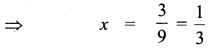# Samacheer Kalvi 11th Maths Solutions Chapter 2 Basic Algebra Ex 2.1

## Tamilnadu Samacheer Kalvi 11th Maths Solutions Chapter 2 Basic Algebra Ex 2.1

Question 1.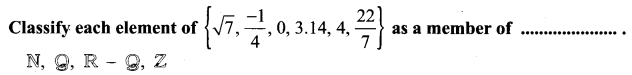Solution: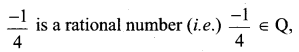3.14 ∈ Q
0, 4 are integers and 0 ∈ Z, 4 ∈ N, Z, Q
$$\frac{22}{7} \in \mathrm{Q}$$Question 2.
Prove that $$\sqrt{3}$$ is an irrational number.
(Hint: Follow the method that we have used to prove $$\sqrt{2}$$ ∉ Q.
Solution:
Suppose that $$\sqrt{3}$$ is rational P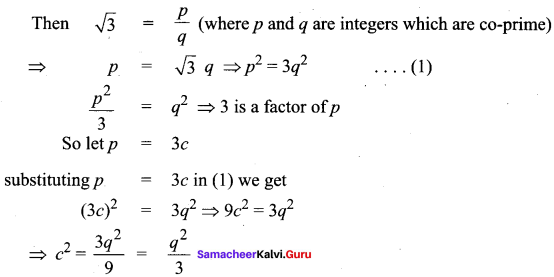⇒ 3 is a factor of q also
so 3 is a factor ofp and q which is a contradiction.
⇒ $$\sqrt{3}$$ is not a rational number
⇒ $$\sqrt{3}$$ is an irrational number

Question 3.
Are there two distinct irrational numbers such that their difference is a rational number? Justify.
Solution:
Taking two irrational numbers as 3 + $$\sqrt{2}$$ and 1 + $$\sqrt{2}$$
Their difference is a rational number. But if we take two irrational numbers as 2 – $$\sqrt{3}$$ and 4 + $$\sqrt{7}$$.
Their difference is again an irrational number. So unless we know the two irrational numbers we cannot say that their difference is a rational number or irrational number.Question 4.
Find two irrational numbers such that their sum is a rational number. Can you find two irrational numbers whose product is a rational number.
Solution:
(i) Let the two irrational numbers as 2 + $$\sqrt{3}$$ and 3 – $$\sqrt{3}$$
Their sum is 2 + $$\sqrt{3}$$ + 3 – 3$$\sqrt{3}$$ which is a rational number.
But the sum of 3 + $$\sqrt{5}$$ and 4 – $$\sqrt{7}$$ is not a rational number. So the sum of two irrational numbers is either rational or irrational.

(ii) Again taking two irrational numbers as π and $$\frac{3}{\pi}$$ their product is $$\sqrt{3}$$ and $$\sqrt{2}$$ = $$\sqrt{3}$$ × $$\sqrt{2}$$ which is irrational, So the product of two irrational numbers is either rational or irrational.

Question 5.
Find a positive number smaller than $$\frac{1}{2^{1000}}$$. Justify.
Solution: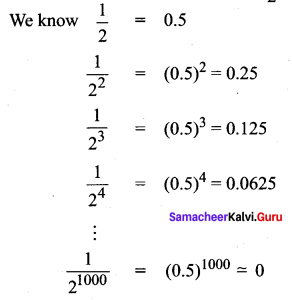There will not be a positive number smaller than 0.
So there will not be a +ve number smaller than $$\frac{1}{2^{1000}}$$

### Samacheer Kalvi 11th Maths Solutions Chapter 2 Basic Algebra Ex 2.1 Additional Questions

Question 1.
Prove that $$\sqrt{5}$$ is an irrational number.
Solution:
Suppose that $$\sqrt{5}$$ is rational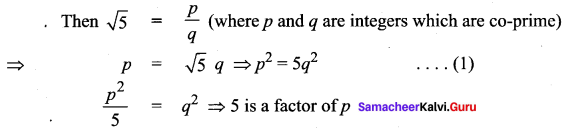So let p = 5c
substitute p = 5c in (1) we get
(5c)2 = 5q2 ⇒ 25c2 = 5q2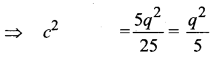⇒ 5 is a factor of q also
So 5 is a factor of p and q which is a contradiction.
⇒ $$\sqrt{5}$$ is not a rational number
⇒ $$\sqrt{5}$$ is an irrational numberQuestion 2.
Prove that 0.33333 = $$\frac{1}{3}$$
Solution:
Let x = 0.33333….
10x = 3.3333 ….
10x – x = 9x = 3